Next: 2 The Characteristic Length Up: J.E. Sonier's Ph.D. Thesis Previous: List of Figures

# 1 Introduction

When a small magnetic field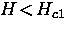is applied to a type-II superconductor, the field is expelled from the interior of the sample--while in an applied magnetic field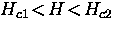it becomes energetically favourable for the field to penetrate the sample in the form of quantized flux lines, called vortices. In the first case (the Meissner state'') the supercurrents which shield the inside of the superconductor from the magnetic field flow around the perimeter of the sample, whereas in the latter case (the vortex state'') shielding currents circulate around the individual vortices.

In the Meissner state, there is some penetration of magnetic field into the surface of the superconductor where the shielding currents circulate. In particular, the magnetic field decays from the surface into the superconductor over a characteristic length scale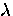, called the magnetic penetration depth''.

In the vortex state, the superconducting carriers which make up the shielding currents circulate faster near the vortex axis. Beyond a critical velocity, superconductivity is destroyed. The region of normal state material near the vortex axis defines the core''. The local magnetic field has a maximum in the center of the vortex core and decays outside the core over the length scale. If the vortices are spaced at a distance much greater thanapart, the field decays to zero--otherwise the field is finite everywhere in the superconductor. The density of the superconducting carriers [or order parameter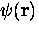] is zero at the vortex center and rises to its maximum value over a distance of the order of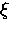, which is called the coherence length''.

Together,andrepresent the characteristic length scales of superconductivity. In particular,is proportional to the density of superconducting carriers. Measurements of this quantity provide a means of investigating the spectrum of low-lying excitations in the superconducting state. On the other hand,is the shortest distance over which the density of superconducting carriers can vary appreciably. Put another way,is the characteristic length scale of the superconducting order parameter.A complete understanding of the behaviour of these parameters (i.e. as a function of temperature, impurities and magnetic field) is an essential ingredient of any theory which attempts to explain the mechanism responsible for superconductivity in the high-Tc materials.

The behaviour ofandis generally considered to be the same in both the Meissner and vortex phases. However, when measuring these length scales, several complications arise from the presence of vortices. At low magnetic fields in the vortex state, the vortex cores are essentially isolated. In this case, one might expect that measurements ofin the vortex state should show the same variation with temperature T and magnetic field H as measurements ofin the Meissner state. However, at higher magnetic fields the vortices interact appreciably with one another, changing the distribution of magnetic field between them. Measurements ofas a function of temperature or magnetic field are sensitive to the way in which the field distribution between the vortices changes. In particular, the function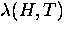which is obtained from experiment depends on the way in which these changes are modelled. Thus in general,obtained from measurements in the vortex state cannot be directly compared toobtained from measurements in the Meissner state.

A second important complication is that the interior of a vortex has an electronic structure which changes with temperature and magnetic field. This electronic structure is a unique property of the vortex and need not be like the quasiparticle states in the normal phase. The precise nature of this electronic structure is still a matter of debate, especially in the high-Tc materials. In these materials the normal state exhibits many anomalous properties, so there is good reason to expect that the electronic structure of the vortex cores is different than that in conventional superconductors.

In the vortex state, the order parameteris diminished appreciably (from its bulk value) in the region of a vortex core. Sinceis the characteristic length for variations in, thenis related to the size of the vortex cores. In a conventional superconductor, the radius of a vortex core r0 is about the size of. Because of the close relationship between r0 andin the vortex state, the terms vortex-core radius'' and coherence length'' will be used interchangeably throughout this thesis. However,should not be confused with the coherence length in the Meissner state. For instance, the size of a vortex core changes as its electronic structure changes. As a result, the variation ofwith temperature and magnetic field in the vortex state is directly linked to the vortex cores. Thus, there is no reason to expect that measurements of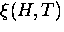in the vortex state will exhibit the same behaviour asin the Meissner phase.

The behaviour ofandis expected to be sensitive to the symmetry of the pairing state. Their behaviour as a function of temperature and magnetic field can be used to resolve the structure of the energy gap in a superconductor. For instance, it is now widely believed that the pairing state in the high-Tc superconductors is one possessing dx2-y2 symmetry, which has four nodal lines on the Fermi surface. The presence of these nodes means that the superconducting state is more sensitive to perturbations than a conventional superconductor, which has an energy gap everywhere on the Fermi surface. In particular, this sensitivity will appear as anomalous changes in the temperature and field dependence of, which is related to the fraction of the superfluid component.

Measurements ofand(i.e. r0) in the vortex state using muon spin rotation (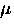SR) spectroscopy is the primary focus of this thesis. What is really measured is the magnetic field distribution in the bulk of the superconductor, which is predominantly due to the vortex lattice. Extractingandrequires some modelling of this distribution. Generally speaking, the current theoretical models are inadequate to fully describe this field distribution. Nevertheless, the data can be fit very well with the field distributions predicted from basic models in which the characteristic length scales are defined. Deviations in the measured behaviour of these length scales, from that predicted by the theory used to model the data, can be attributed to a problem with the definition ofandin the theory itself. In this sense, what is really reported in this thesis is an effective'' penetration depth and an effective'' coherence length.

I will begin with a basic introduction to the characteristic length scales as they pertain to conventional and unconventional superconductors. I shall proceed to discuss how theSR technique can be used to obtain bothand r0 (which is closely related to the coherence length), from the measured internal field distribution. Currently, this technique is the only way to studyin the bulk'' of the high-Tc cuprates, deep in the superconducting state.

I shall then explain how one can model the measured internal field distribution. The effects of pinning and thermal fluctuations will be discussed as they pertain to both a 3D and a 2D vortex lattice. This will be followed by an outline of the current theoretical description of the vortex structure in both conventional s-wave and unconventional d-wave superconductors.

Next, I shall present measurements of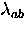and r0 in the conventional superconductor NbSe2, and in the optimally doped and underdoped high-Tc superconductors YBa2Cu3O6.95 and YBa2Cu3O6.60, respectively. The results are taken from our most recent work which appears in a series of short papers [2,3,4,5]. We shall find thatexhibits unconventional behaviour as a function of both temperature and magnetic field in YBa2Cu3O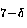.I also show that r0 changes dramatically as a function of temperature and field, and that the strength of these variations differs markedly in YBa2Cu3Ofrom what is observed in NbSe2. I shall show that the qualitative behaviour of the extracted values ofand r0 is fairly robust with respect to the way in which theSR data is modelled. I conclude by summarizing and discussing the novel features associated with the measurements in YBa2Cu3Orelative to those of NbSe2.Next: 2 The Characteristic Length Up: J.E. Sonier's Ph.D. Thesis Previous: List of Figures